Скачать презентацию Magnetism A Whole New Topic March 5 2007

• Количество слайдов: 75Magnetism A Whole New Topic March 5, 2007 Magnetism 1This Week n n n Exam #2 Returned Today we begin magnetism – a topic that will occupy us for most of the remainder of the semester. Quiz on Friday Watch for Web. Assignment Next week: Spring Break – Enjoy! Magnetism 2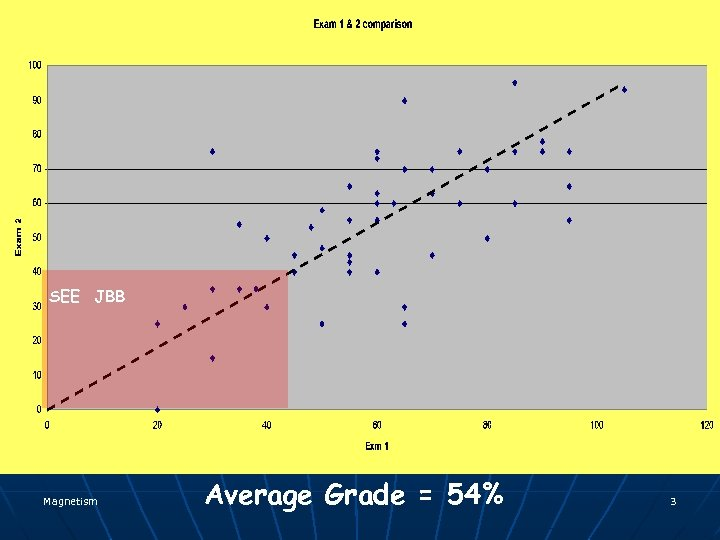SEE JBB Magnetism Average Grade = 54% 3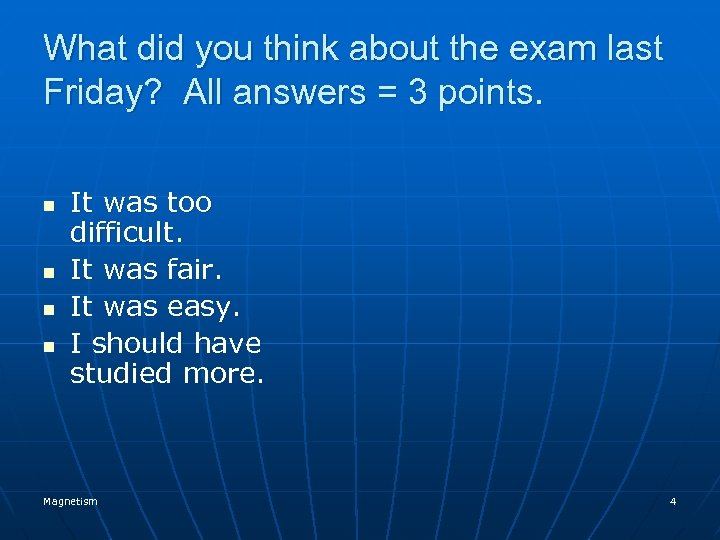What did you think about the exam last Friday? All answers = 3 points. n n It was too difficult. It was fair. It was easy. I should have studied more. Magnetism 4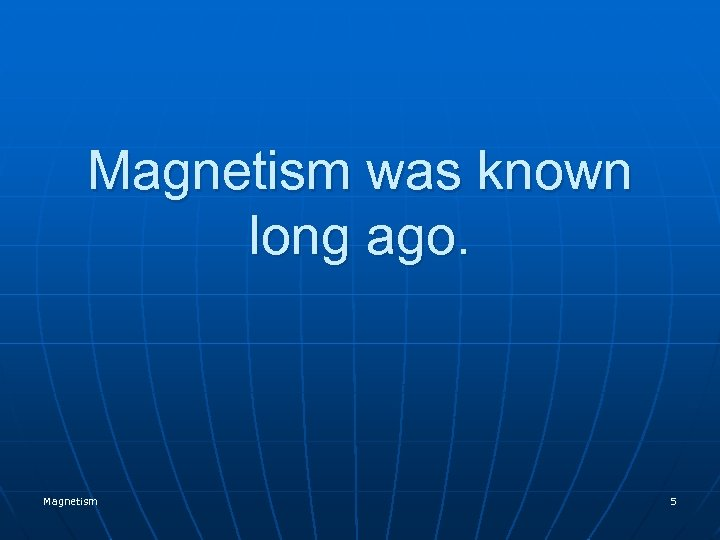Magnetism was known long ago. Magnetism 5Lodestone (Mineral) • Lodestones attracted iron filings. • Lodestones seemed to attract each other. • Lodestone is a natural magnet. Magnetism 6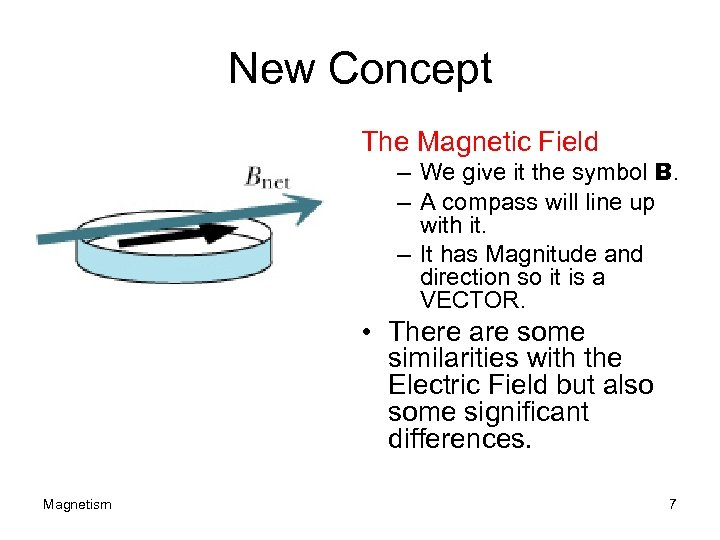New Concept The Magnetic Field – We give it the symbol B. – A compass will line up with it. – It has Magnitude and direction so it is a VECTOR. • There are some similarities with the Electric Field but also some significant differences. Magnetism 7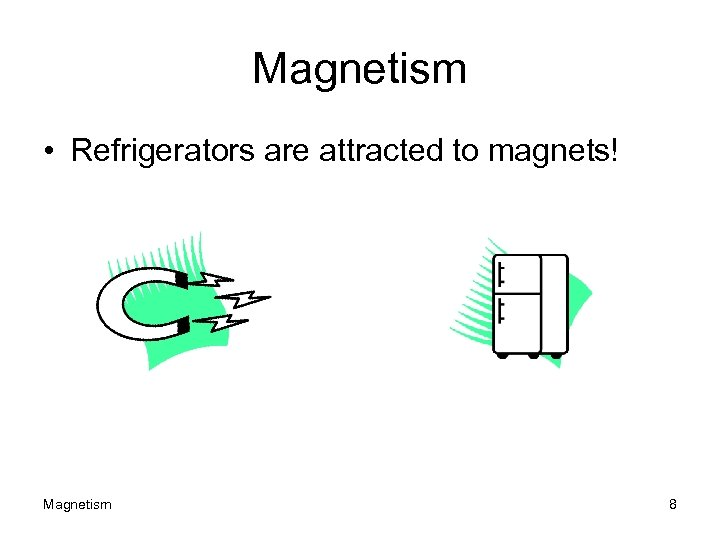Magnetism • Refrigerators are attracted to magnets! Magnetism 8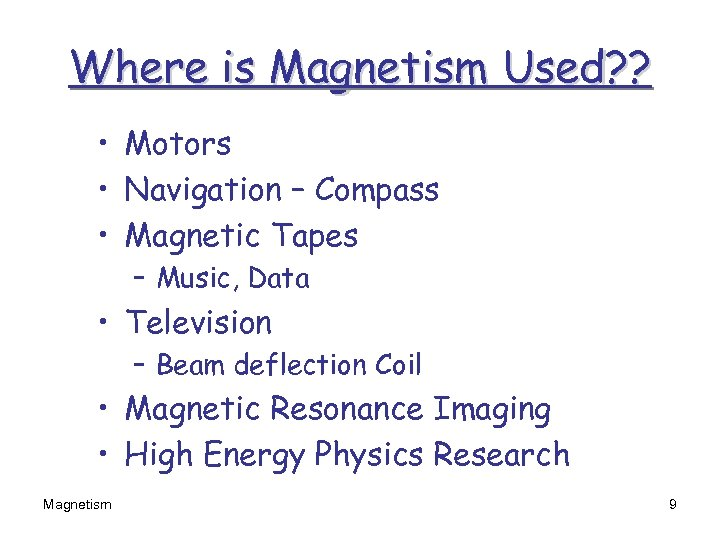Where is Magnetism Used? ? • Motors • Navigation – Compass • Magnetic Tapes – Music, Data • Television – Beam deflection Coil • Magnetic Resonance Imaging • High Energy Physics Research Magnetism 9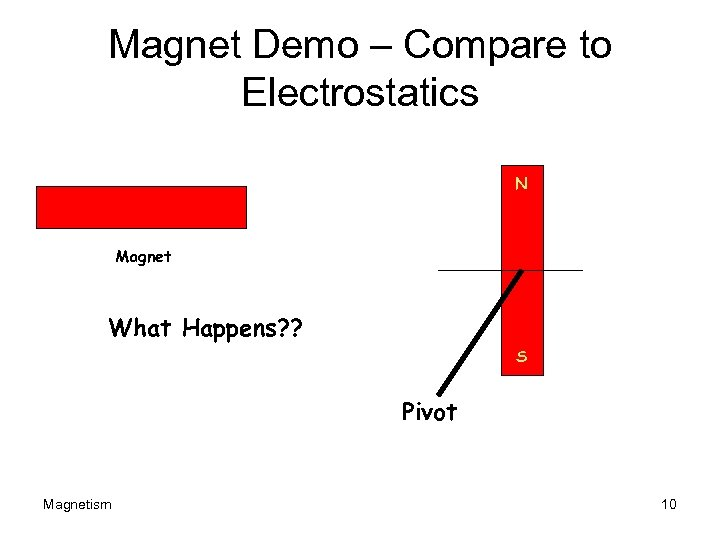Magnet Demo – Compare to Electrostatics N Magnet What Happens? ? S Pivot Magnetism 10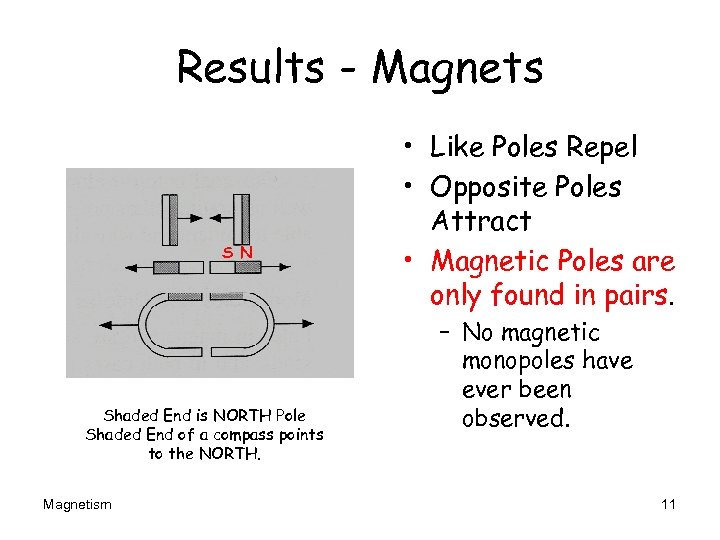Results - Magnets S N Shaded End is NORTH Pole Shaded End of a compass points to the NORTH. Magnetism • Like Poles Repel • Opposite Poles Attract • Magnetic Poles are only found in pairs. – No magnetic monopoles have ever been observed. 11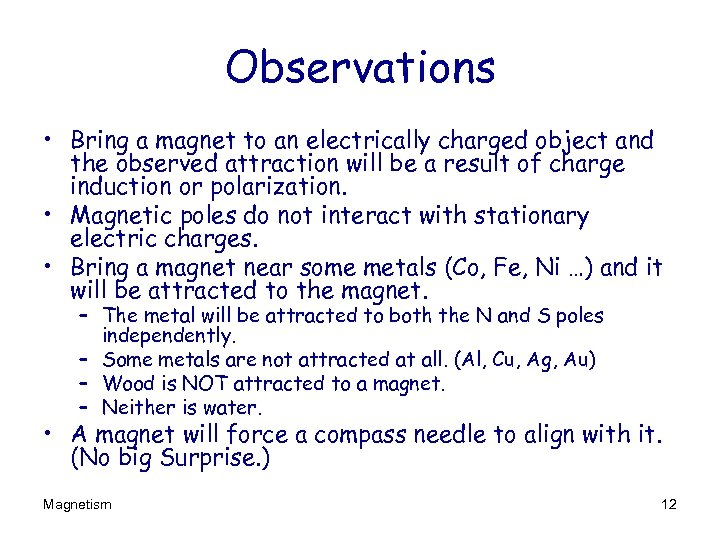Observations • Bring a magnet to an electrically charged object and the observed attraction will be a result of charge induction or polarization. • Magnetic poles do not interact with stationary electric charges. • Bring a magnet near some metals (Co, Fe, Ni …) and it will be attracted to the magnet. – The metal will be attracted to both the N and S poles independently. – Some metals are not attracted at all. (Al, Cu, Ag, Au) – Wood is NOT attracted to a magnet. – Neither is water. • A magnet will force a compass needle to align with it. (No big Surprise. ) Magnetism 12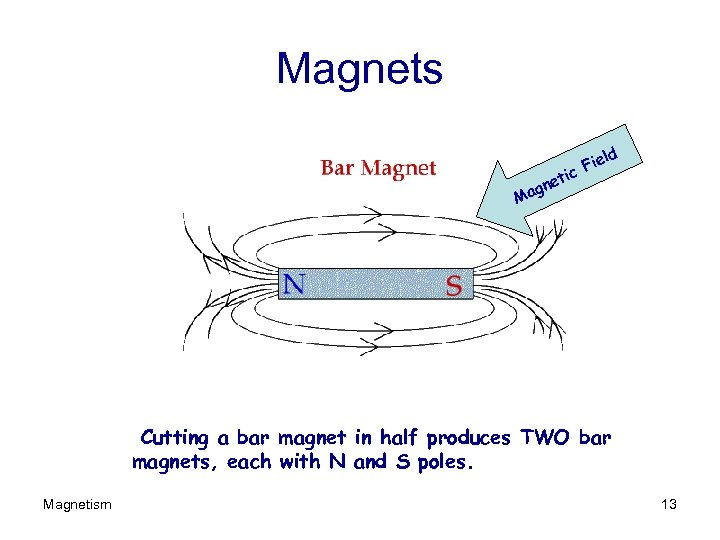Magnets ld ie c. F ti gne Ma Cutting a bar magnet in half produces TWO bar magnets, each with N and S poles. Magnetism 13Consider a Permanent Magnet N S The magnetic Field B goes from North to South. Magnetism 14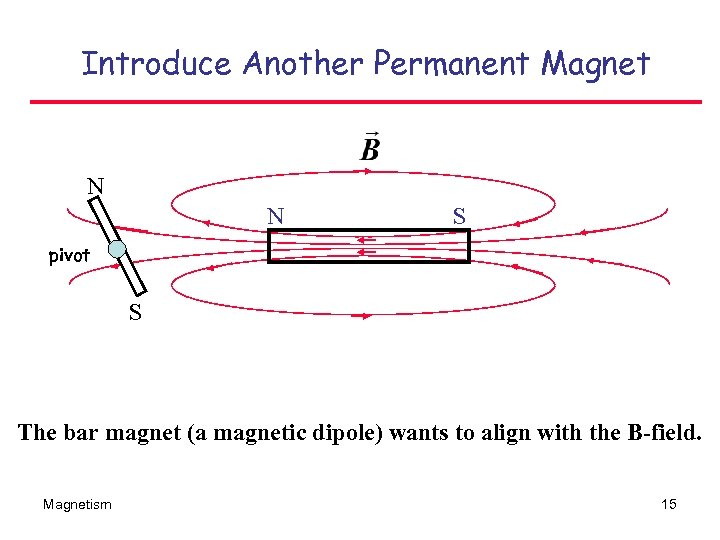Introduce Another Permanent Magnet N N S pivot S The bar magnet (a magnetic dipole) wants to align with the B-field. Magnetism 15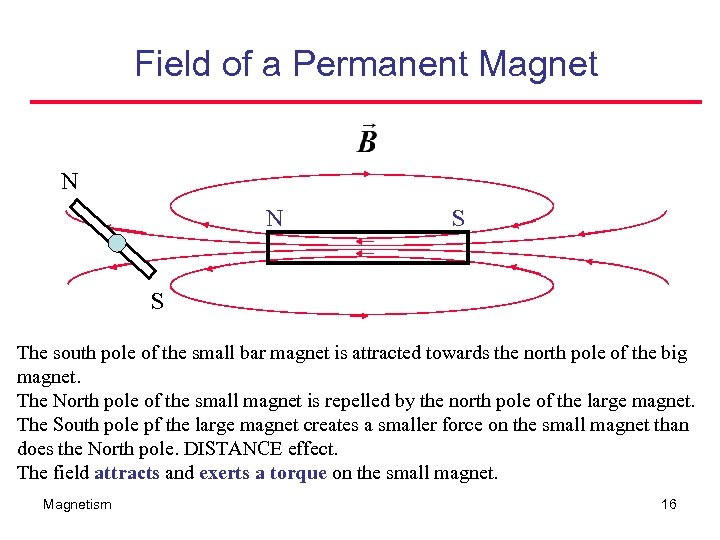Field of a Permanent Magnet N N S S The south pole of the small bar magnet is attracted towards the north pole of the big magnet. The North pole of the small magnet is repelled by the north pole of the large magnet. The South pole pf the large magnet creates a smaller force on the small magnet than does the North pole. DISTANCE effect. The field attracts and exerts a torque on the small magnet. Magnetism 16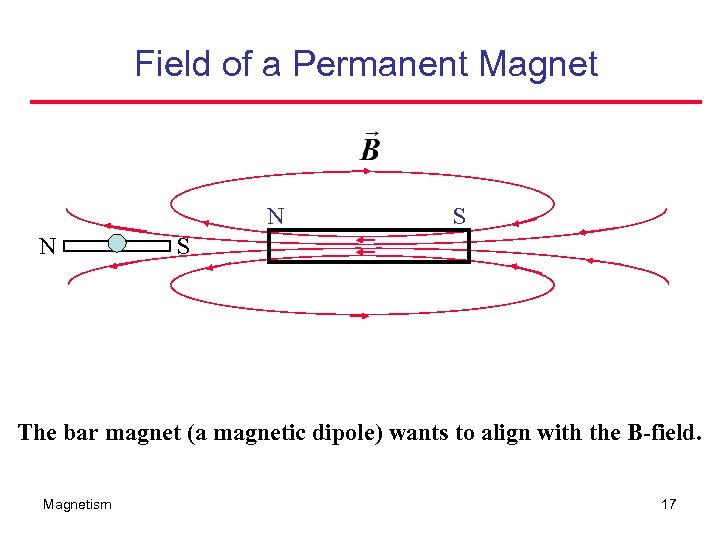Field of a Permanent Magnet N N S S The bar magnet (a magnetic dipole) wants to align with the B-field. Magnetism 17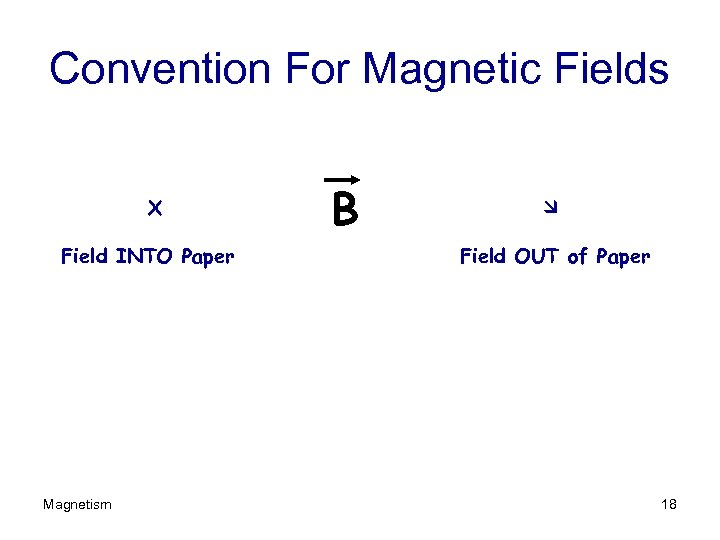Convention For Magnetic Fields X Field INTO Paper Magnetism B Field OUT of Paper 18Typical Representation Magnetism 19Experiments with Magnets Show • Current carrying wire produces a circular magnetic field around it. • Force (actually torque) on a Compass Needle (or magnet) increases with current. Magnetism 20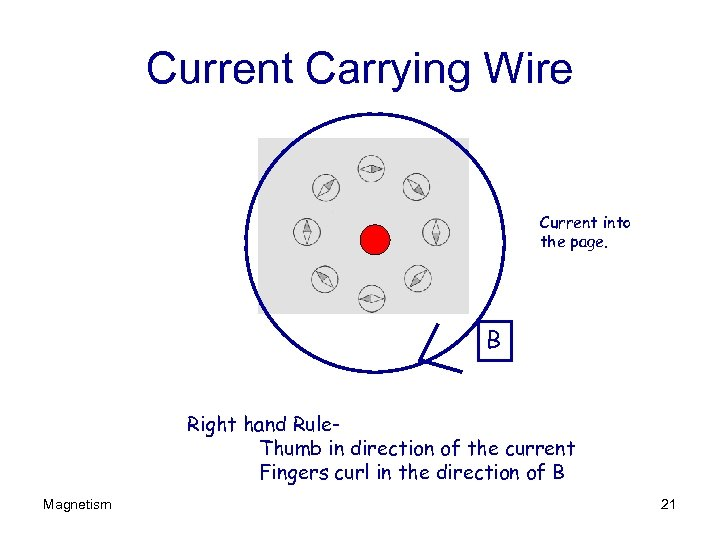Current Carrying Wire Current into the page. B Right hand Rule. Thumb in direction of the current Fingers curl in the direction of B Magnetism 21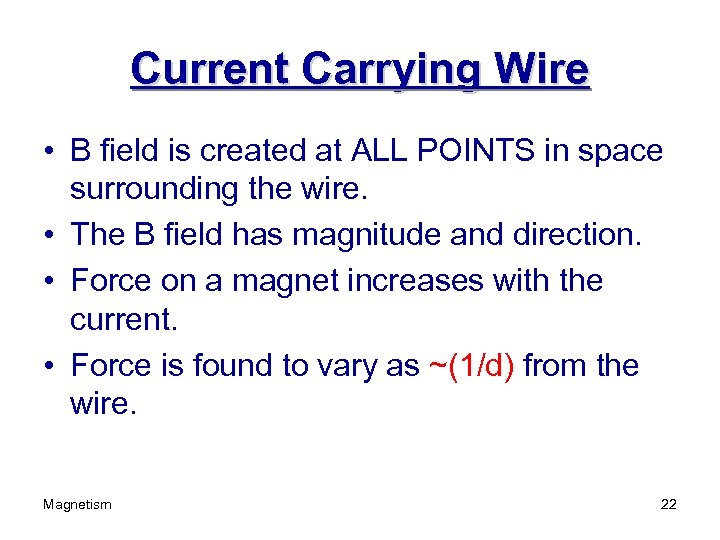Current Carrying Wire • B field is created at ALL POINTS in space surrounding the wire. • The B field has magnitude and direction. • Force on a magnet increases with the current. • Force is found to vary as ~(1/d) from the wire. Magnetism 22Compass and B Field • Observations – North Pole of magnets tend to move toward the direction of B while S pole goes the other way. – Field exerts a TORQUE on a compass needle. – Compass needle is a magnetic dipole. – North Pole of compass points toward the NORTH. Magnetism 23Planet Earth Magnetism 24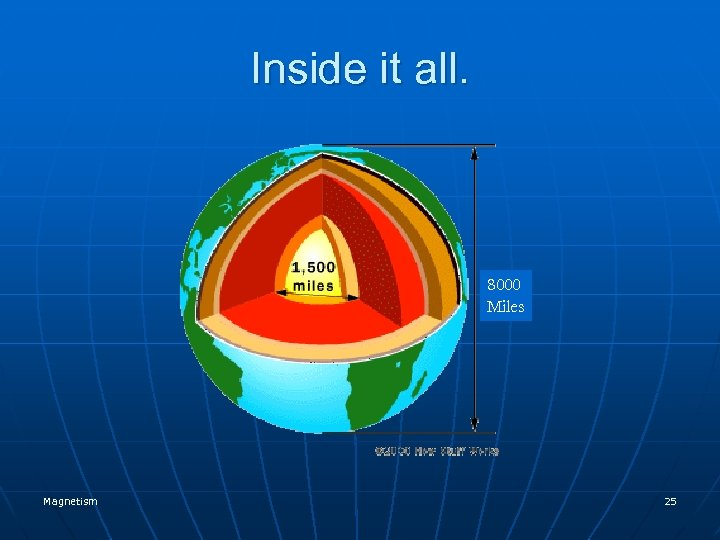Inside it all. 8000 Miles Magnetism 25On the surface it looks like this. . Magnetism 26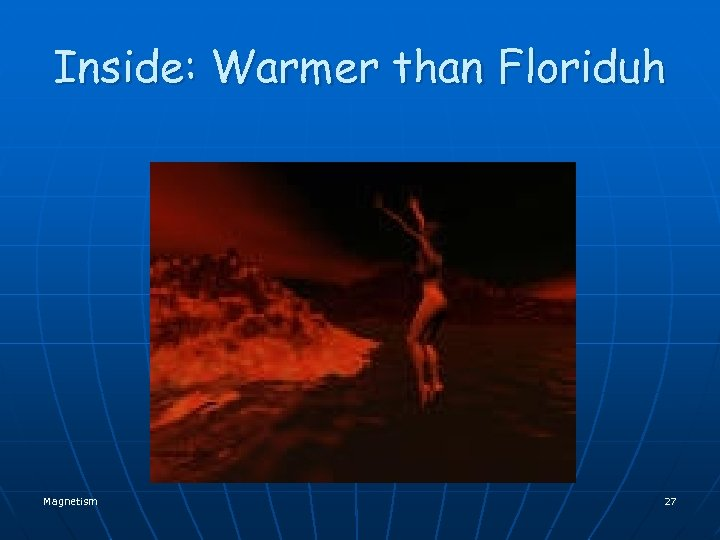Inside: Warmer than Floriduh Magnetism 27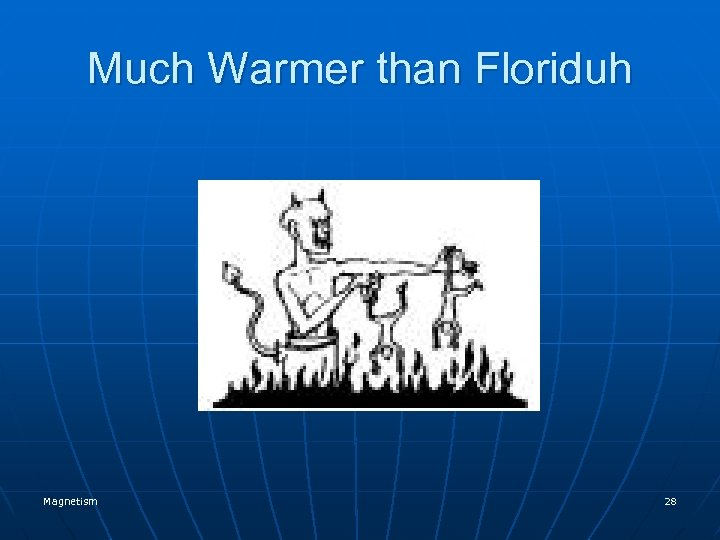Much Warmer than Floriduh Magnetism 28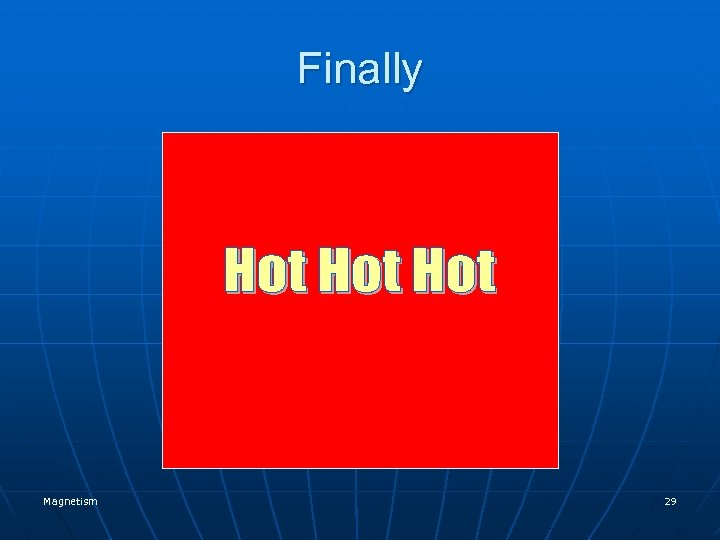Finally Magnetism 29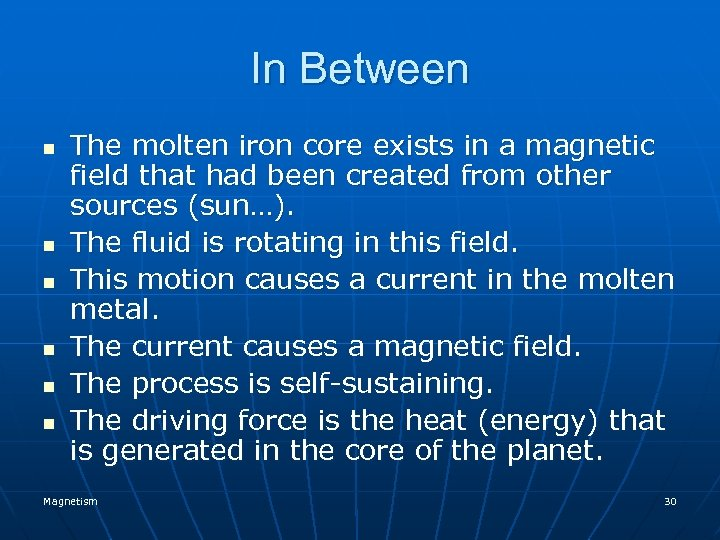In Between n n n The molten iron core exists in a magnetic field that had been created from other sources (sun…). The fluid is rotating in this field. This motion causes a current in the molten metal. The current causes a magnetic field. The process is self-sustaining. The driving force is the heat (energy) that is generated in the core of the planet. Magnetism 30After molten lava emerges from a volcano, it solidifies to a rock. In most cases it is a black rock known as basalt, which is faintly magnetic, like iron emerging from a melt. Its magnetization is in the direction of the local magnetic force at the time when it cools down. Instruments can measure the magnetization of basalt. Therefore, if a volcano has produced many lava flows over a past period, scientists can analyze the magnetizations of the various flows and from them get an idea on how the direction of the local Earth's field varied in the past. Surprisingly, this procedure suggested that times existed when the magnetization had the opposite direction from today's. All sorts of explanation were proposed, but in the end the only one which passed all tests was that in the distant past, indeed, the magnetic polarity of the Earth was sometimes reversed. Magnetism 31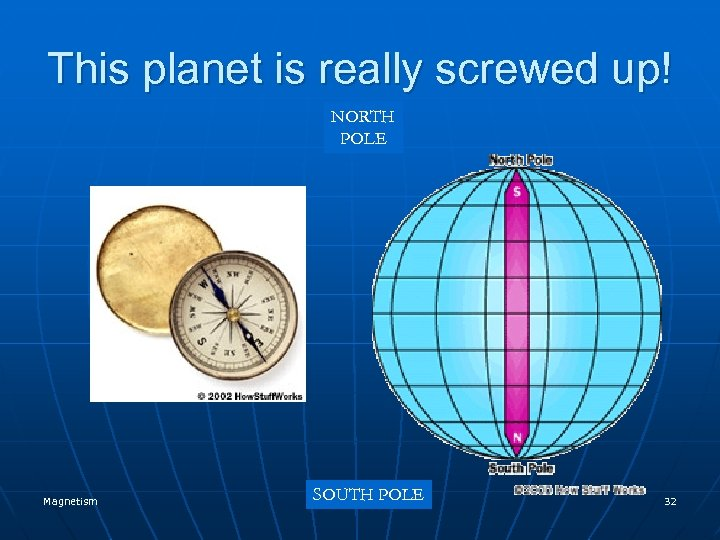This planet is really screwed up! NORTH POLE Magnetism SOUTH POLE 32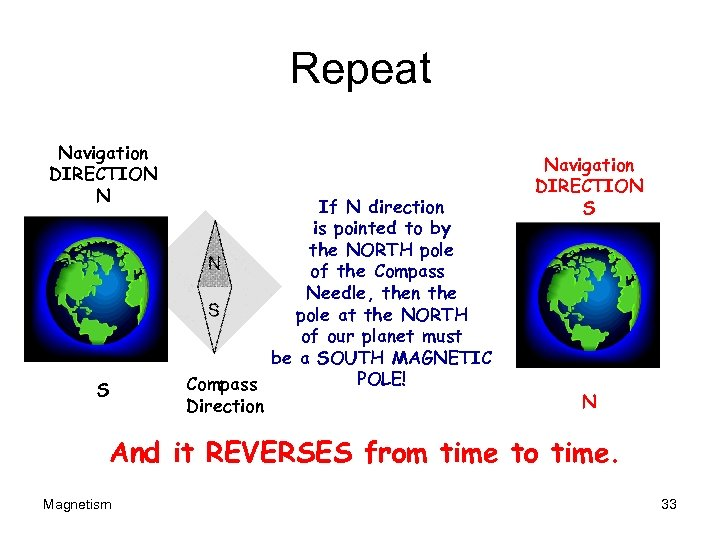Repeat Navigation DIRECTION N S If N direction is pointed to by the NORTH pole of the Compass Needle, then the pole at the NORTH of our planet must be a SOUTH MAGNETIC POLE! Compass Direction Navigation DIRECTION S N And it REVERSES from time to time. Magnetism 33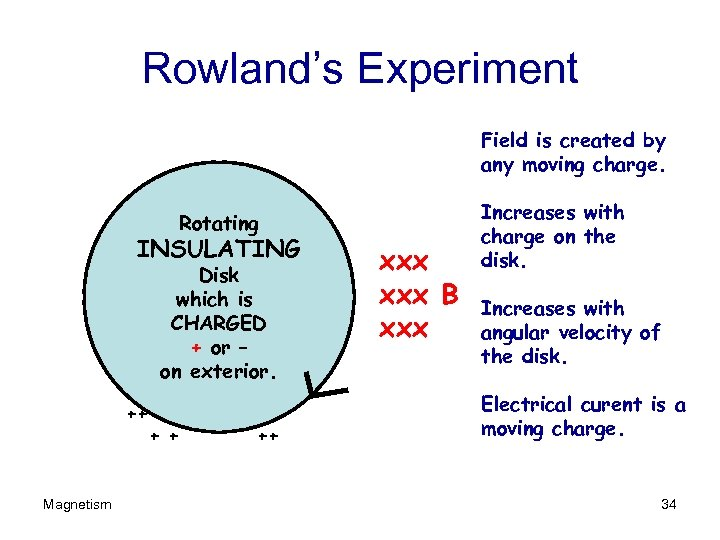Rowland’s Experiment Field is created by any moving charge. Rotating INSULATING Disk which is CHARGED + or – on exterior. ++ Magnetism + + ++ xxx B xxx Increases with charge on the disk. Increases with angular velocity of the disk. Electrical curent is a moving charge. 34A Look at the Physics q There is NO force on a charge placed into a magnetic field if the charge is NOT moving. There is no force if the charge moves parallel to the field. q • If the charge is moving, there is a force on the charge, perpendicular to both v and B. F = q v x B Magnetism 35WHAT THE HECK IS THAT? ? ? • A WHAT PRODUCT? • A CROSS PRODUCT – Like an angry one? ? • Alas, yes …. • F=qv X B Magnetism 36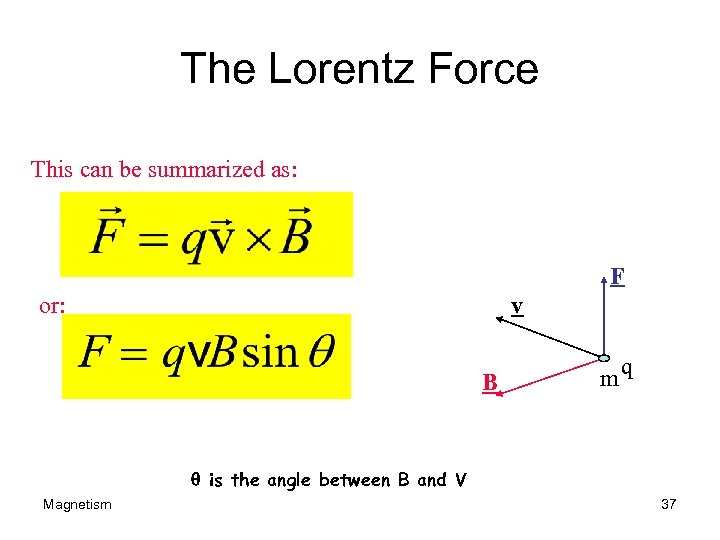The Lorentz Force This can be summarized as: F or: v B mq q is the angle between B and V Magnetism 37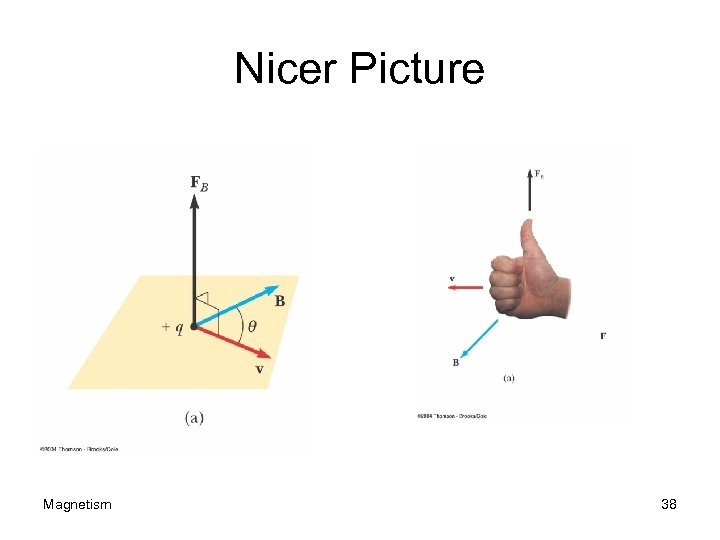Nicer Picture Magnetism 38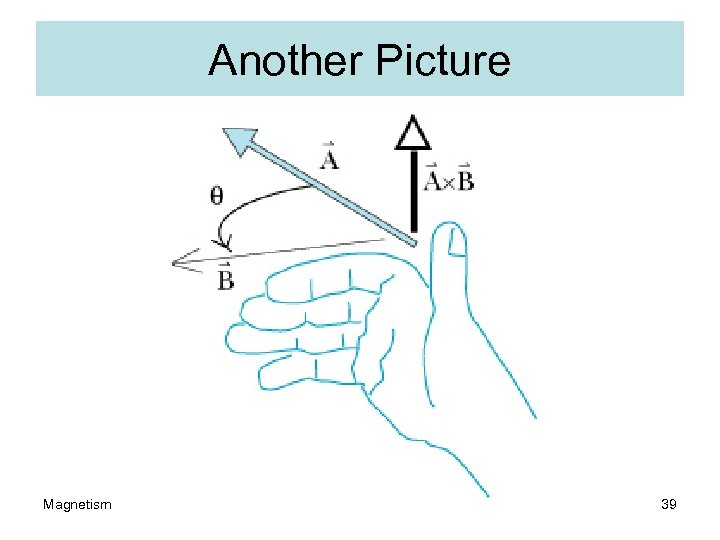Another Picture Magnetism 39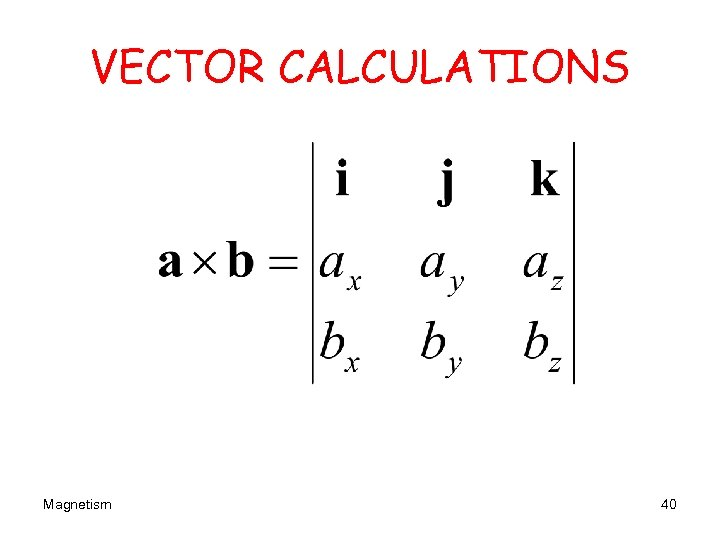VECTOR CALCULATIONS Magnetism 40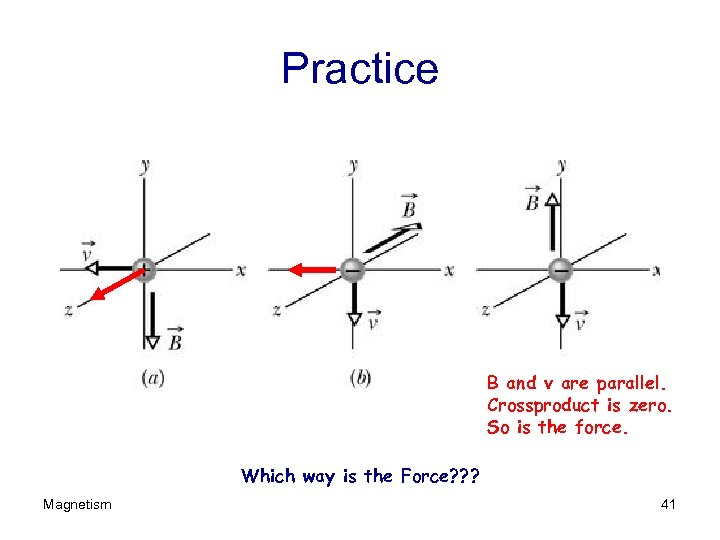Practice B and v are parallel. Crossproduct is zero. So is the force. Which way is the Force? ? ? Magnetism 41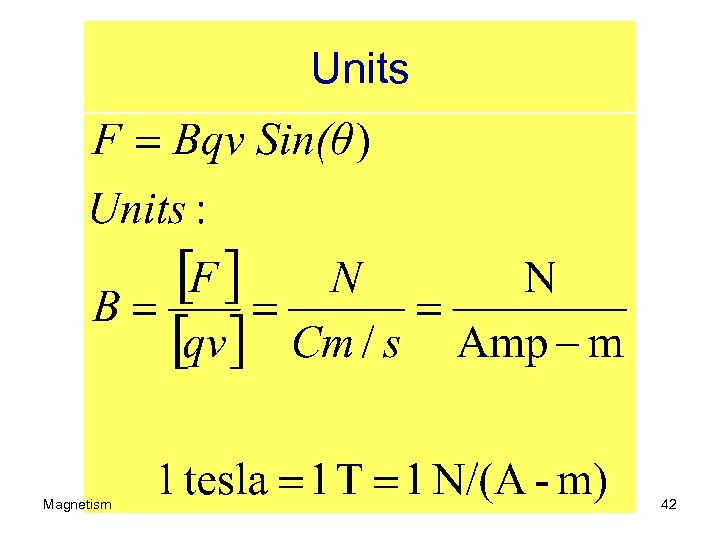Units Magnetism 42teslas are Magnetism 43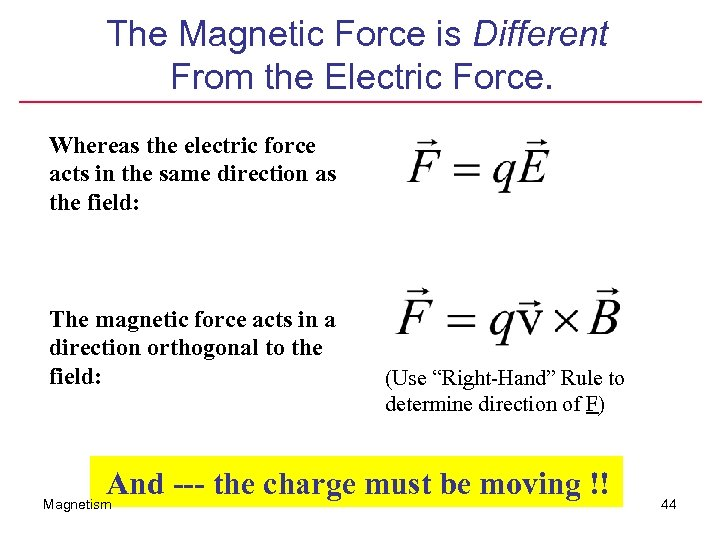The Magnetic Force is Different From the Electric Force. Whereas the electric force acts in the same direction as the field: The magnetic force acts in a direction orthogonal to the field: (Use “Right-Hand” Rule to determine direction of F) And --- the charge must be moving !! Magnetism 44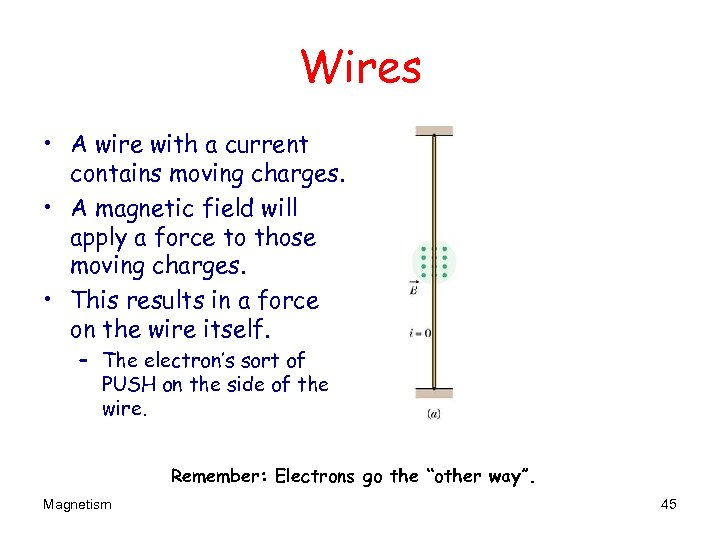Wires • A wire with a current contains moving charges. • A magnetic field will apply a force to those moving charges. • This results in a force on the wire itself. – The electron’s sort of PUSH on the side of the wire. F Remember: Electrons go the “other way”. Magnetism 45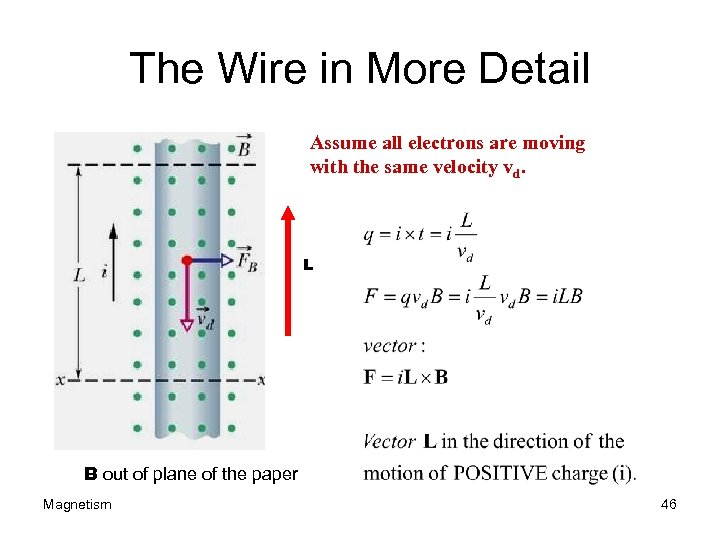The Wire in More Detail Assume all electrons are moving with the same velocity vd. L B out of plane of the paper Magnetism 46Magnetic Levitation Magnetic Force mg Where does B point? ? Magnetism Current = i Into the paper. 47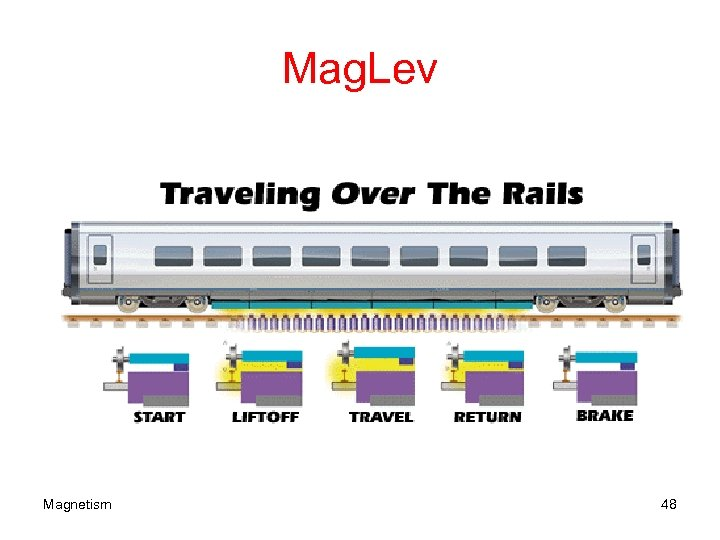Mag. Lev Magnetism 48A conductor suspended by two flexible wires as shown in the diagram has a mass per unit length of 0. 040 0 kg/m. What current must exist in the conductor in order for the tension in the supporting wires to be zero when the magnetic field is 3. 60 T into the page? What is the required direction for the current? Concrete Insulator Magnetism 49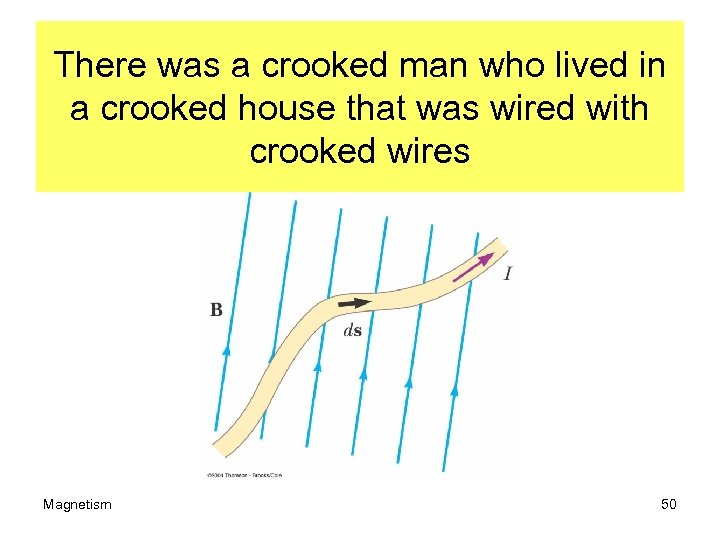There was a crooked man who lived in a crooked house that was wired with crooked wires Magnetism 50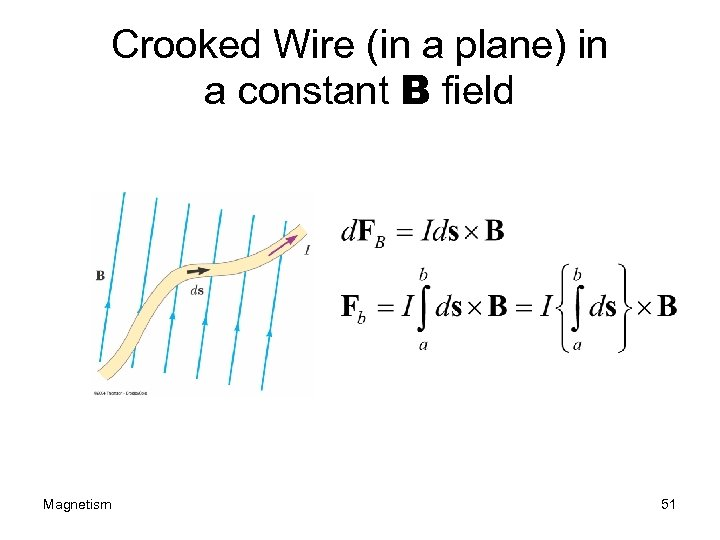Crooked Wire (in a plane) in a constant B field Magnetism 51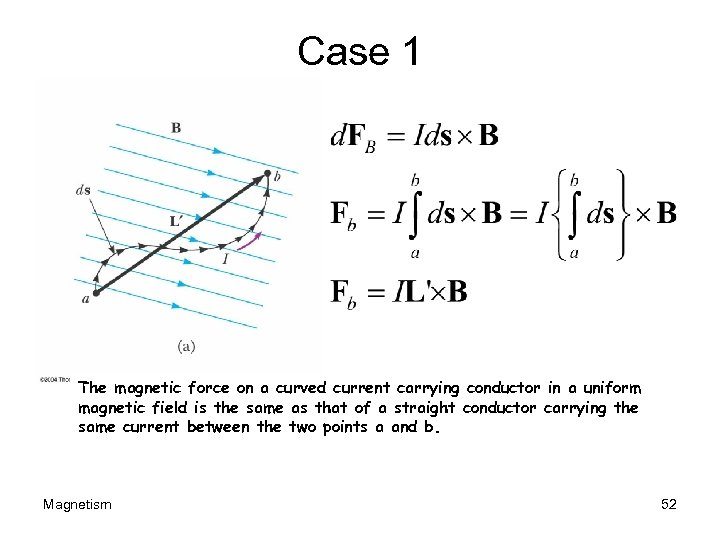Case 1 The magnetic force on a curved current carrying conductor in a uniform magnetic field is the same as that of a straight conductor carrying the same current between the two points a and b. Magnetism 52Case 2 The net magnetic force on a closed current carrying loop is ZERO! Magnetism 53Current Loop What is force on the ends? ? Loop will tend to rotate due to the torque the field applies to the loop. Magnetism 54The Loop (From the top) OBSERVATION Force on Side 2 is out of the paper and that on the opposite side is into the paper. No net force tending to rotate the loop due to either of these forces. The net force on the loop is also zero, pivot Magnetism 55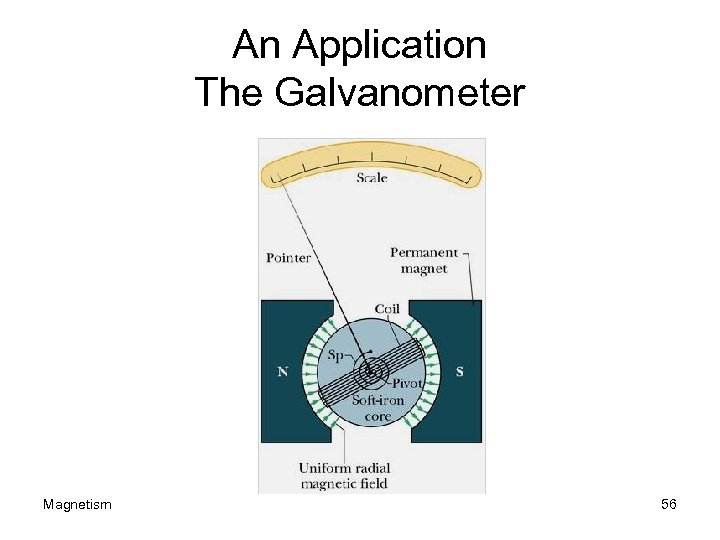An Application The Galvanometer Magnetism 56The other sides t 1=F 1 (b/2)Sin(q) =(B i a) x (b/2)Sin(q) total torque on the loop is: 2 t 1 Total torque: t=(ia. B) b. Sin(q) =i. ABSin(q) (A=Area) Magnetism 57A Coil Normal to the coil RIGHT HAND RULE TO FIND NORMAL TO THE COIL: “Point or curl you’re the fingers of your right hand in the direction of the current and your thumb will point in the direction of the normal to the coil. Magnetism 58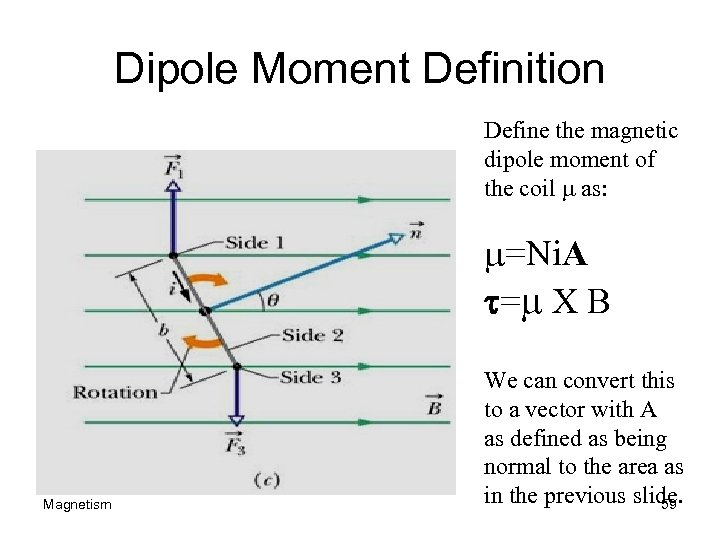Dipole Moment Definition Define the magnetic dipole moment of the coil m as: m=Ni. A t=m X B Magnetism We can convert this to a vector with A as defined as being normal to the area as in the previous slide. 59A 40. 0 -cm length of wire carries a current of 20. 0 A. It is bent into a loop and placed with its normal perpendicular to a magnetic field with a magnitude of 0. 520 T. What is the torque on the loop if it is bent into (a)an equilateral triangle? (b)What is the torque if the loop is (c) a square or (d) a circle? (e) Which torque is greatest? Magnetism 60Motion of a charged particle in a magnetic Field Magnetism 61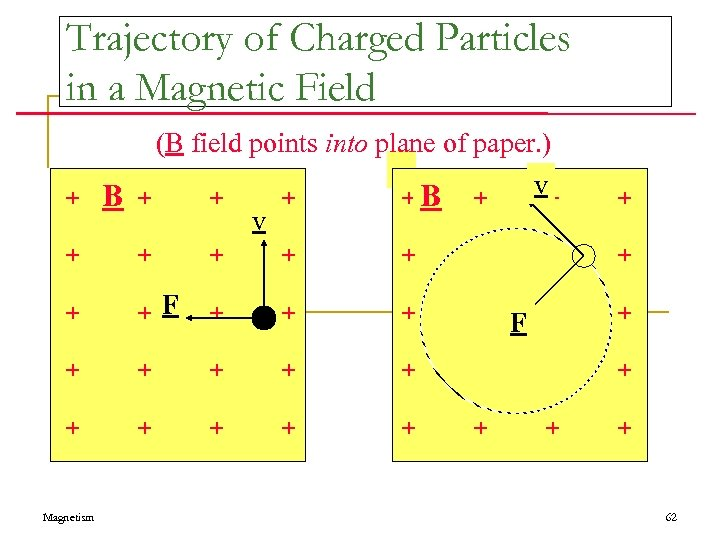Trajectory of Charged Particles in a Magnetic Field (B field points into plane of paper. ) + +B + v+ + + + + F + + + + + + B + + + Magnetism v 62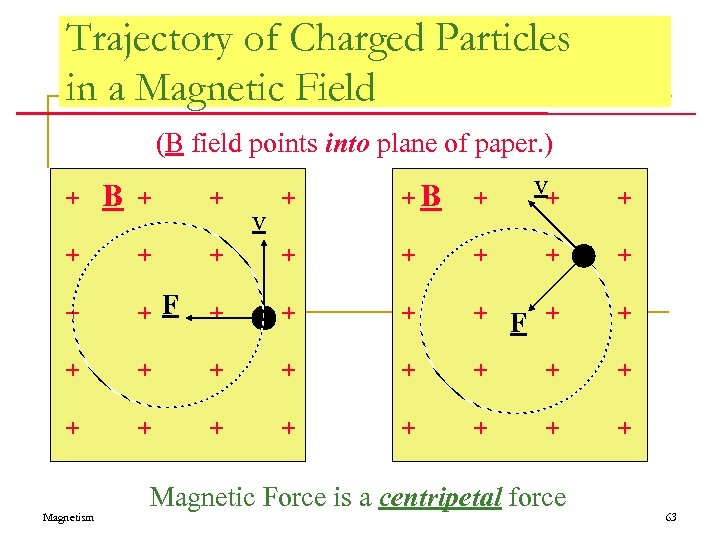Trajectory of Charged Particles in a Magnetic Field (B field points into plane of paper. ) v+ + B + +B + v + + + F + + + + + + Magnetism Magnetic Force is a centripetal force 63Review of Rotational Motion = s / r s = r ds/dt = d /dt r v = r s r = angle, = angular speed, = angular acceleration at ar at = r tangential acceleration ar = v 2 / r radial acceleration The radial acceleration changes the direction of motion, while the tangential acceleration changes the speed. Uniform Circular Motion ar = constant v and ar constant but direction changes v Magnetism ar = v 2/r = 2 r KE = ½ mv 2 = ½ mw 2 r 2 F = mar = mv 2/r = m 2 r 64Magnetism 65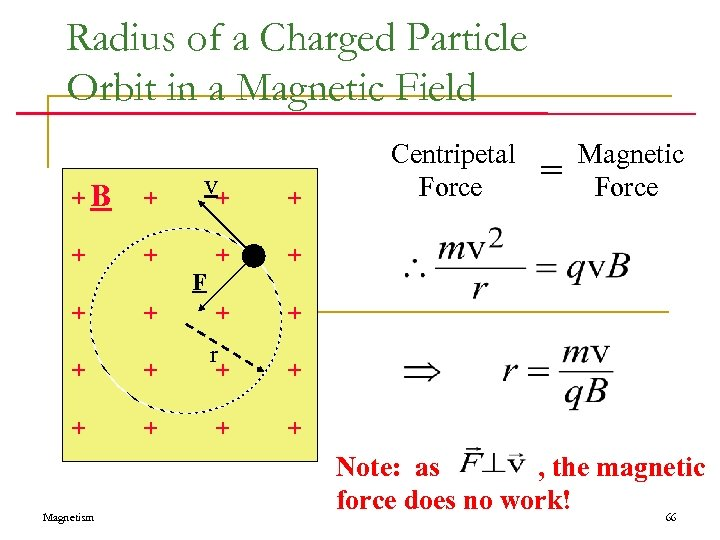Radius of a Charged Particle Orbit in a Magnetic Field +B + v+ + + r + + + Centripetal Magnetic = Force Force + Magnetism F Note: as , the magnetic force does no work! 66Cyclotron Frequency +B + v + + + r + + + The time taken to complete one orbit is: + Magnetism F + 67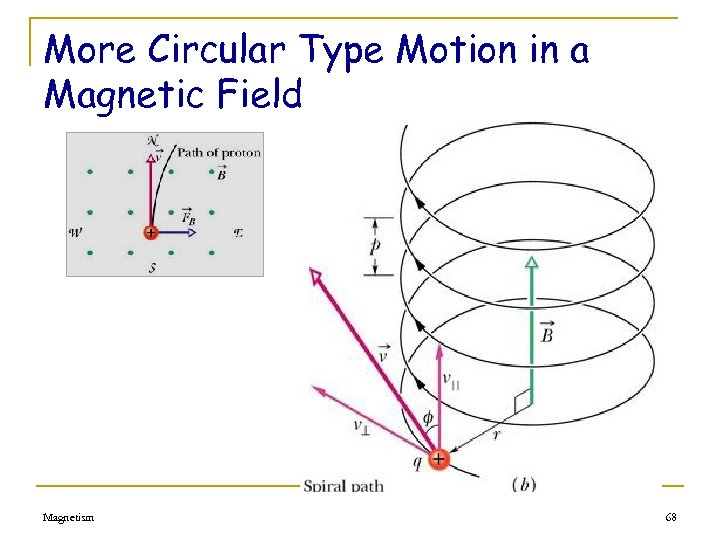More Circular Type Motion in a Magnetic Field Magnetism 68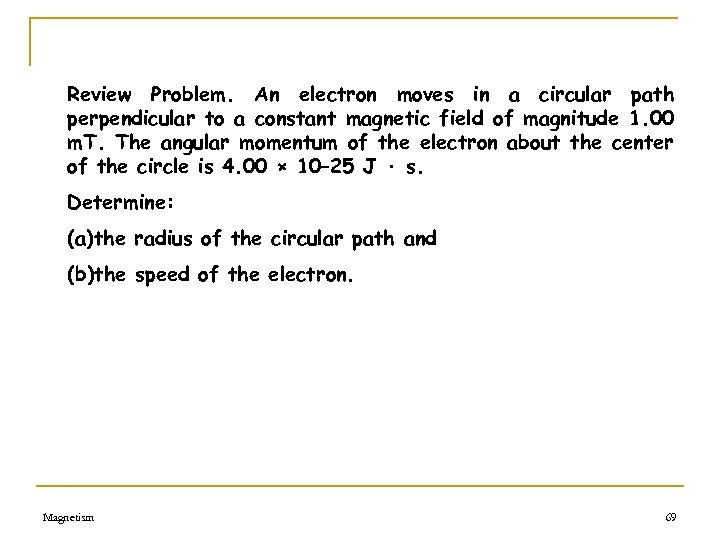Review Problem. An electron moves in a circular path perpendicular to a constant magnetic field of magnitude 1. 00 m. T. The angular momentum of the electron about the center of the circle is 4. 00 × 10– 25 J · s. Determine: (a)the radius of the circular path and (b)the speed of the electron. Magnetism 69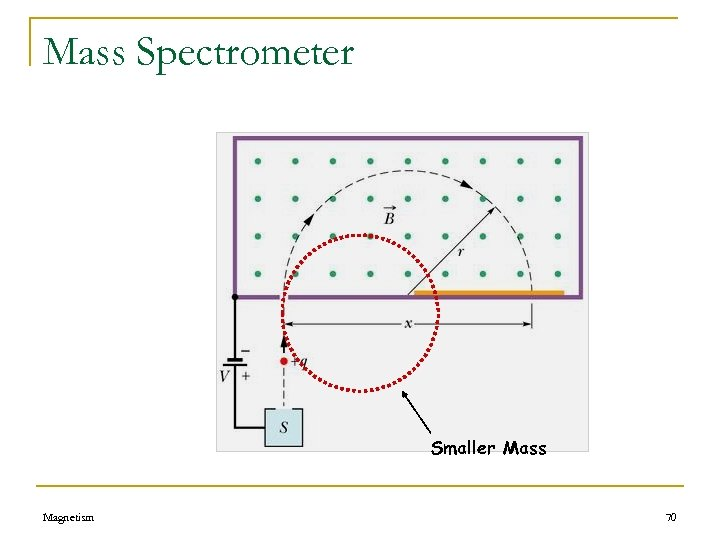Mass Spectrometer Smaller Mass Magnetism 70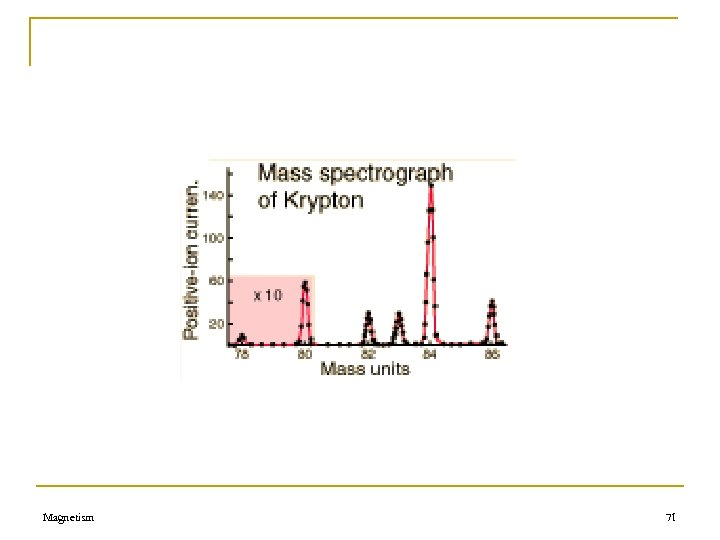Magnetism 71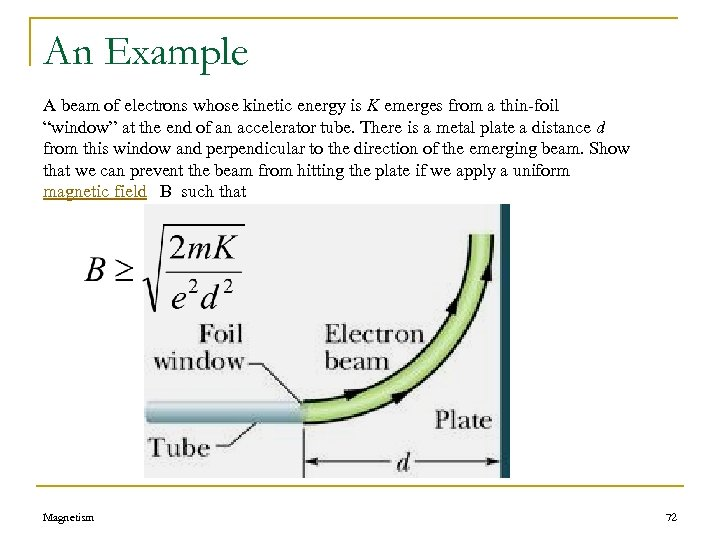An Example A beam of electrons whose kinetic energy is K emerges from a thin-foil “window” at the end of an accelerator tube. There is a metal plate a distance d from this window and perpendicular to the direction of the emerging beam. Show that we can prevent the beam from hitting the plate if we apply a uniform magnetic field B such that Magnetism 72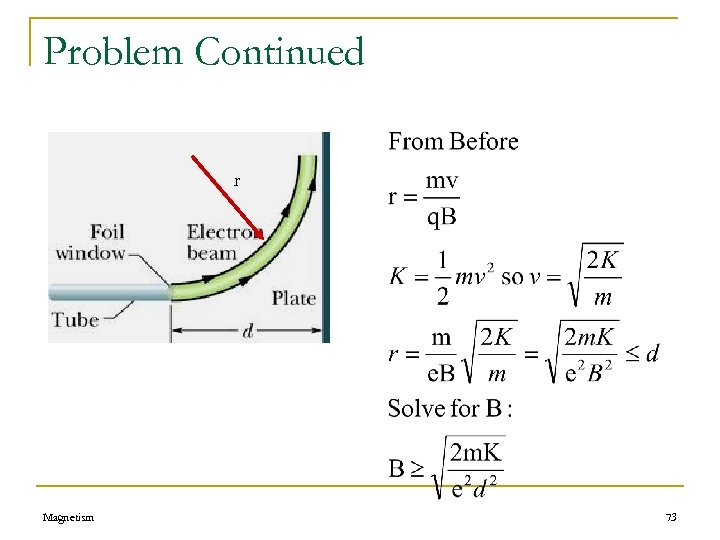Problem Continued r Magnetism 73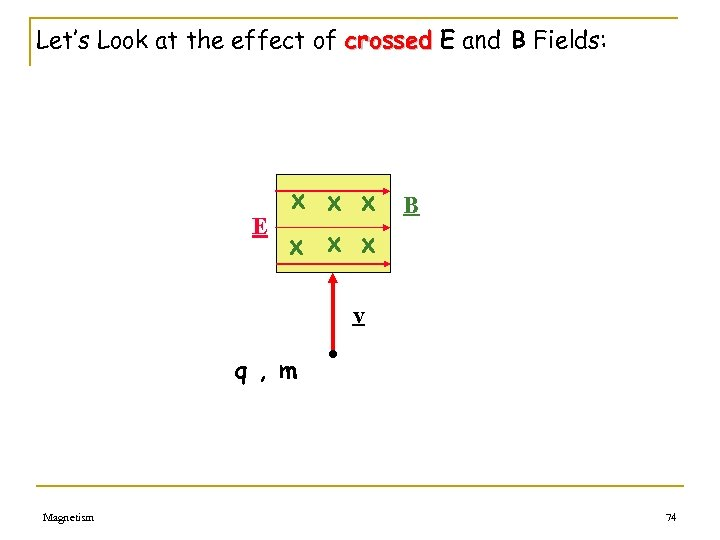Let’s Look at the effect of crossed E and B Fields: x x x B E x x x v q , m Magnetism • 74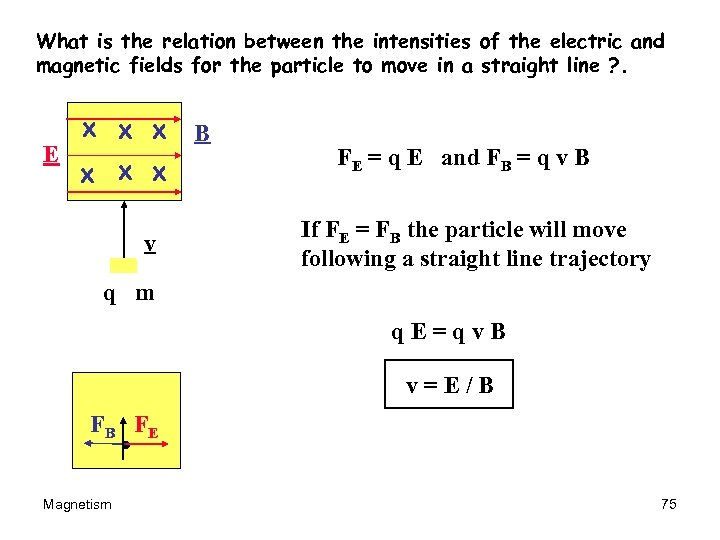What is the relation between the intensities of the electric and magnetic fields for the particle to move in a straight line ? . x x x B E x x x v q • m FE = q E and FB = q v B If FE = FB the particle will move following a straight line trajectory q. E=qv. B v=E/B FB FE • Magnetism 75